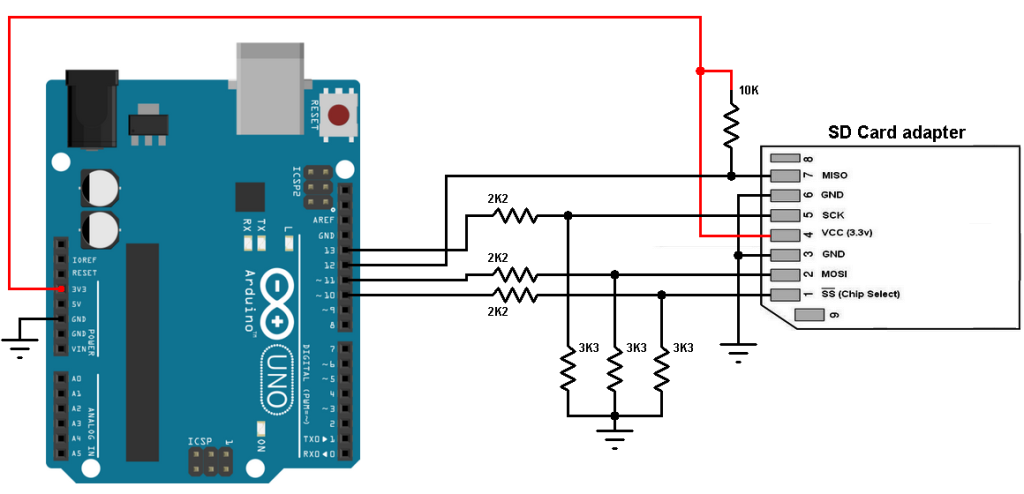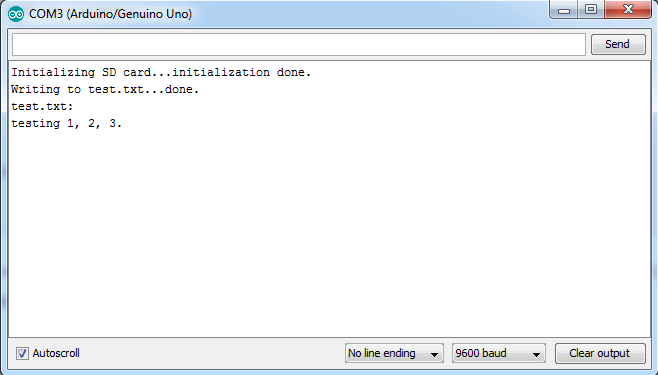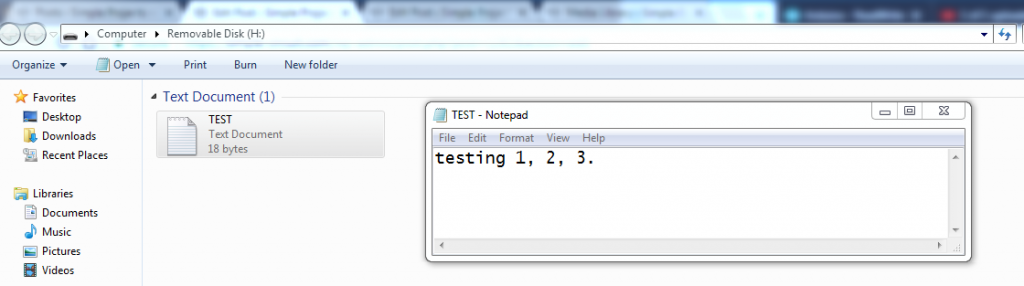# Arduino and SD card example – Read and write files

This example shows how to read and write data to and from an SD card. Example will be tested in a real hardware circuit and simulated with Proteus software.

Last time I tested the simple example which displays SD card information on serial monitor, example link is below:
Arduino and SD card interfacing example

Hardware Required:

• Arduino board
• SD card with FAT16 or FAT32 file system
• SD card socket (connector)
• 10K ohm resistor
• 3 x 3.3K ohm resistor
• 3 x 2.2K ohm resistor
• Jumper wires

The circuit:
Example circuit schematic diagram is shown below.(Grounded terminals are connected together)

The SD card is supplied from the Arduino board with 3.3V.

In the circuit there are 3 voltage dividers, each one consists of 2.2K and 3.3K resistors, they are used to step down 5V that comes from the arduino into 3V which is sufficient for the SD card signals. The voltage dividers are used for SD card signals: SCK (serial clock), MOSI (master out slave in) and SS (chip select). The Arduino sends these signals from pins 13, 11 and 10 respectively. The SD card MISO is connected directly to the arduino because this path is used by the SD card to send data to the arduino (with voltage of 3.3V). The master device is the arduino and the slave device is the SD card.
Connecting the SD card directly to the arduino without voltage level converters or voltage dividers may damage it.

Arduino Code:
I got the code below from arduino examples (with minor modifications), it reads and writes data to and from an SD card connected to the Arduino, results are printed to Arduino IDE serial monitor.

As a result, I placed a samsung 2 GB micro SD card formatted with FAT16 file system and I got the result shown below (Arduino IDE serial monitor):Opening the file with Microsoft windows:Proteus simulation should give the same results as shown in the following video: